Question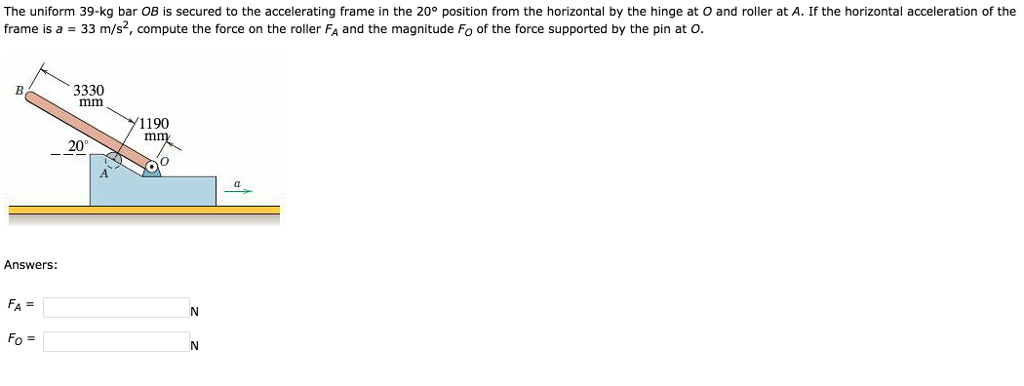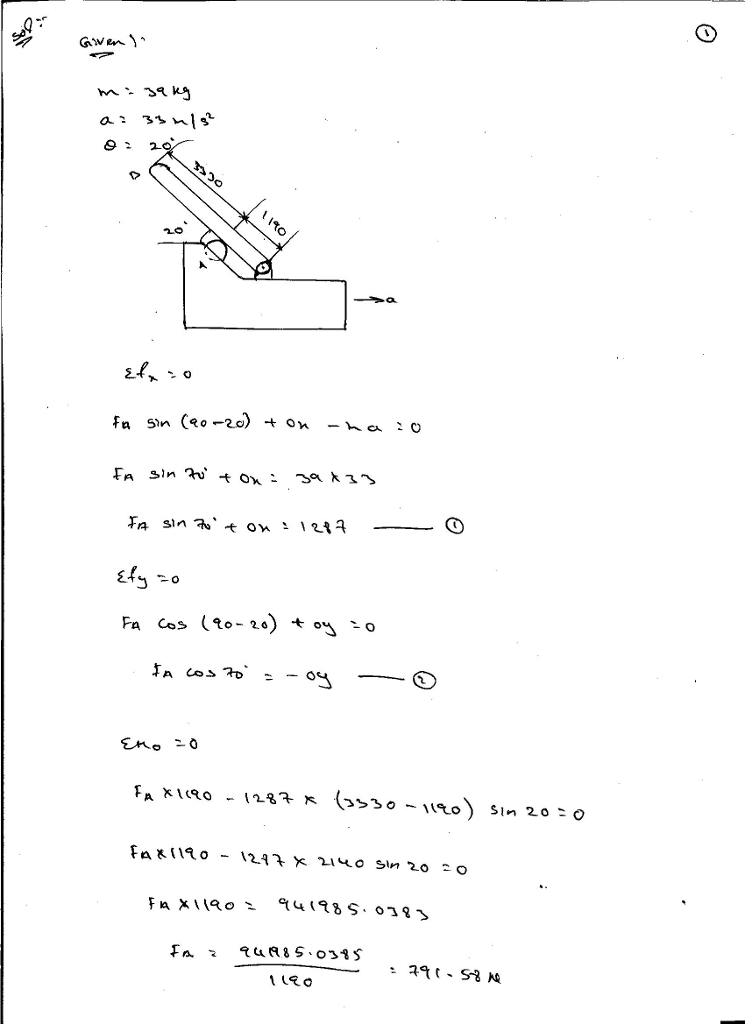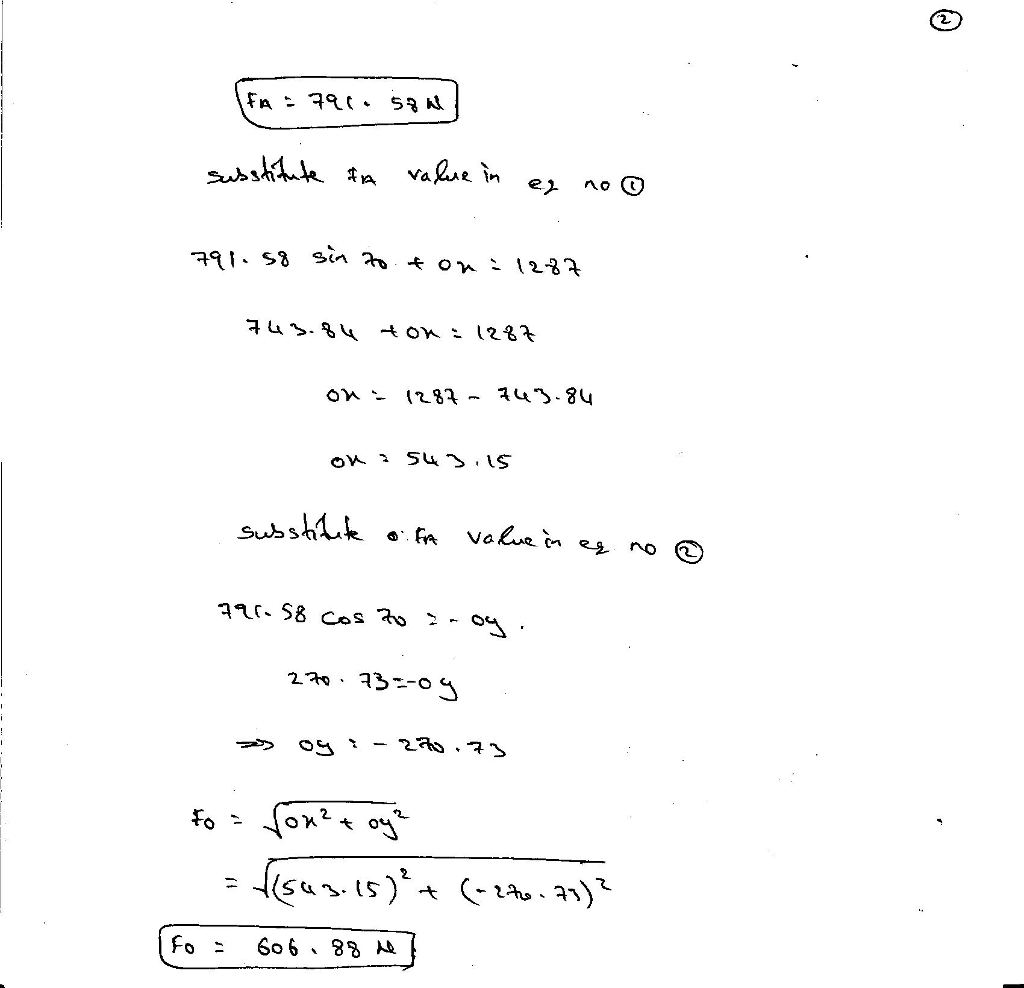#### Earn Coins

Coins can be redeemed for fabulous gifts.

Similar Homework Help Questions
• ### The crank OA rotates in the vertical plane with a constant clockwise angular velocity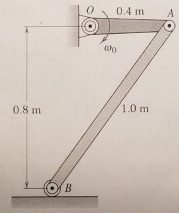The crank OA rotates in the vertical plane with a constant clockwise angular velocity ωo=4.5 rad/s. For the position when OA is horizontal, (a) determine the angular acceleration of the uniform 10 kg slender bar AB and the horizontal and vertical components of the acceleration of its center of mass. Use the methods of kinematics (Ch 16) to establish both the magnitude and direction → or ← for aG and; CW or CCW for αAB) of each variable.  (b) Draw complete free body...

• ### The uniform bar of mass m and length L is balanced in the vertical position when...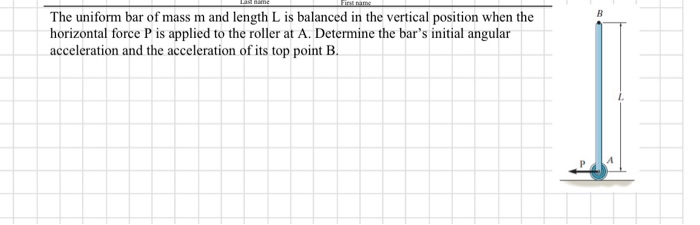The uniform bar of mass m and length L is balanced in the vertical position when the horizontal force P is applied to the roller at A. Determine the bar's ntal angular acceleration and the acceleration of its top point B.

• ### Dont use vector method 5. The uniform 200 kg bar AB is raised in the vertical...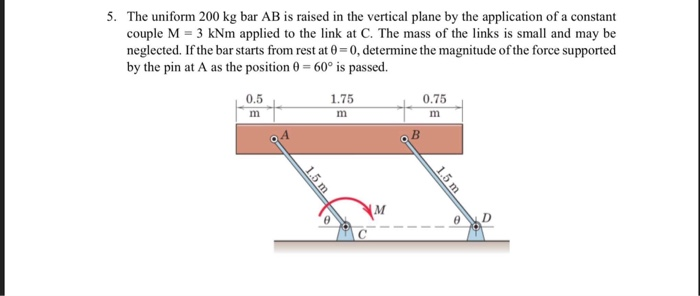Dont use vector method 5. The uniform 200 kg bar AB is raised in the vertical plane by the application of a constant couple M 3 kNm applied to the link at C. The mass of the links is small and may be neglected. If the bar starts from rest at 0-0, determine the magnitude of the force supported by the pin at A as the position 0 600 is passed. 0.5 1.75 0.75

• ### Prob. 3 A slender uniform homogeneous bar of total mass 2 kg is used to stiffen...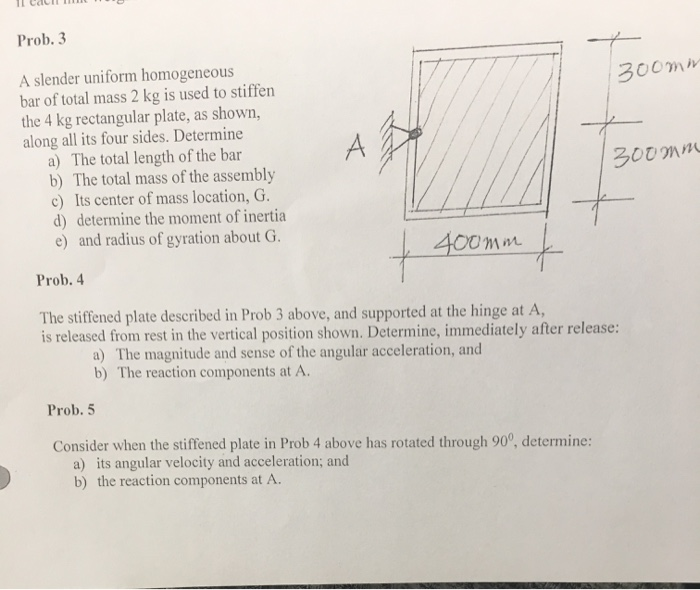Prob. 3 A slender uniform homogeneous bar of total mass 2 kg is used to stiffen the 4 kg rectangular plate, as shown, along all its four sides. Determine a) The total length of the bar b) The total mass of the assembly c) Its center of mass location, G. d) determine the moment of inertia e) and radius of gyration about G. 300mm A 300mm + 400mm Prob. 4 The stiffened plate described in Prob 3 above, and supported...

• ### Uniform bar OA rotates around endpoint O and position angle 0= 0 when the bar is...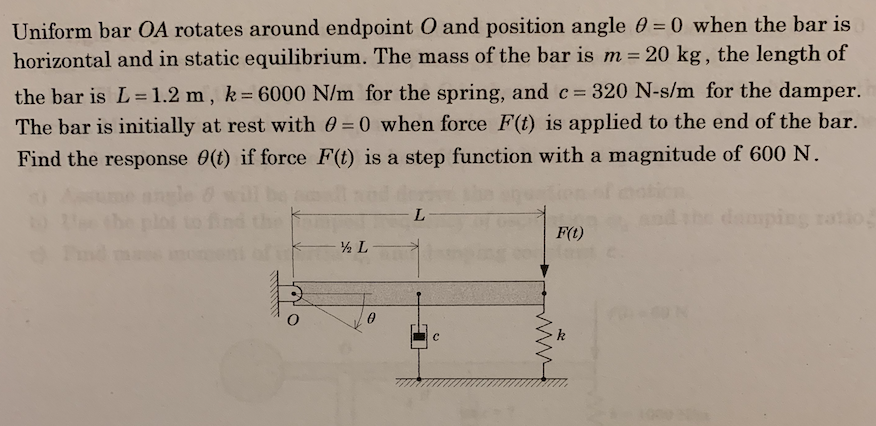Uniform bar OA rotates around endpoint O and position angle 0= 0 when the bar is horizontal and in static equilibrium. The mass of the bar is m = 20 kg, the length of the bar is L=1.2 m , k= 6000 N/m for the spring, and c= 320 N-s/m for the damper. The bar is initially at rest with 0 0 when force F(t) is applied to the end of the bar. Find the response 0(t) if force F(t)...

• ### In Fig. 12-33, one end of a uniform beam of mass 40.0 kg is hinged to...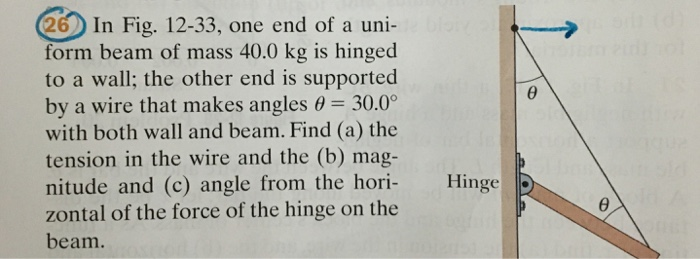In Fig. 12-33, one end of a uniform beam of mass 40.0 kg is hinged to a wall: the other end is supported by a wire that makes angles theta = 30.0degree with both wall and beam. Find the tension in the wire and the magnitude and angle from the horizontal of the force of the hinge on the beam.

• ### The ABC bar has a mass of 20 kg with radius of rotation around its center mass C 150 mm. The bar is secured to the face...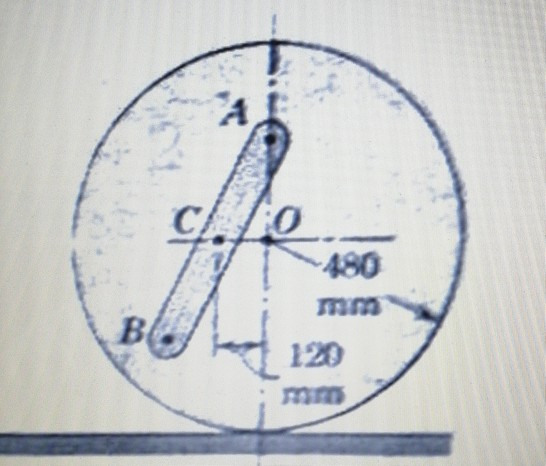The ABC bar has a mass of 20 kg with radius of rotation around its center mass C 150 mm. The bar is secured to the face of a uniform circular disk, which has a mass of 30 kg. If the disk and the rod attached there to are released from rest in the position shown. Calculate the maximum angular velocity of the disk during movement. The disk rolls without sliding. A CAFO 480 mm B 120 A CAFO 480...

• ### The 600 mm long homogeneous slender bar OA has a mass of 8 kg and is supported in the horizontal position by the cable at A and the corner hinge at O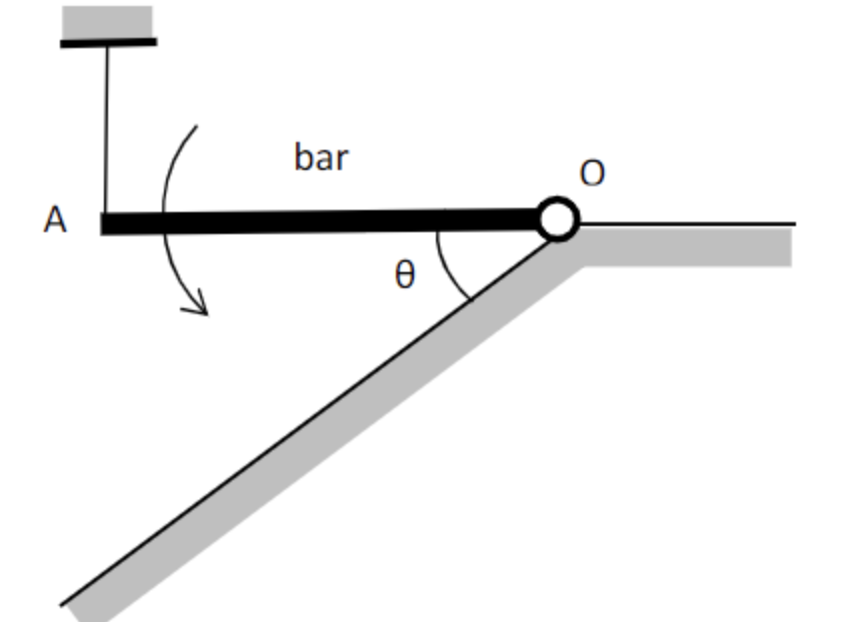The 600 mm long homogeneous slender bar OA has a mass of 8 kg and is supported in the horizontal position by the cable at A and the corner hinge at O. The cable at A is suddenly released. Just before hitting the incline, the bar has an angular velocity of ω= 5 rad / sec. Calculate the angle θ. Neglect the curvature of the path of the bar mass center when the bar is falling and assume that the...

• ### The 10 kg uniform slender bar is pinned at A to the collar, which has an upward acceleration a = 4 m/s along the vertical shaft.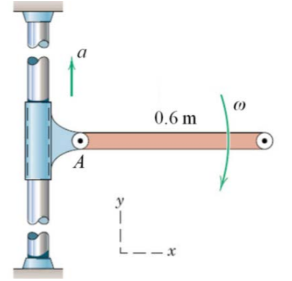The 10 kg uniform slender bar is pinned at A to the collar, which has an upward acceleration a = 4 m/s along the vertical shaft. If the bar has an angular velocity ω = 3 rad/s as it swings past the horizontal position, determine the magnitude of the reaction at A for this instant.Ans: 43.8 N

• ### (30 Pints) The 10-kg bar is released from the horizontal position when it is rested. The...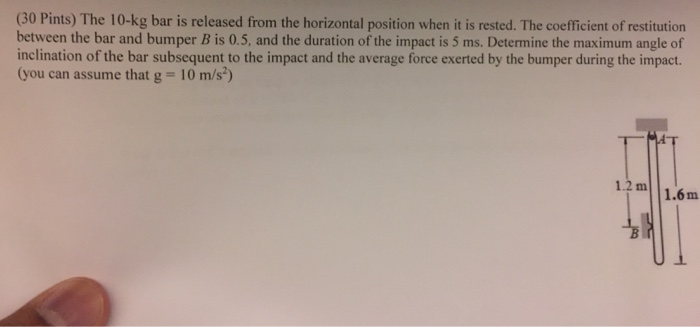(30 Pints) The 10-kg bar is released from the horizontal position when it is rested. The coefficient of restitution between the bar and bumper B is 0.5, and the duration of the impact is 5 ms. Determine the maximum angle of inclination of the bar subsequent to the impact and the average force exerted by the bumper during the impact. (you can assume that g 10 m/s?) 12 m 1.6m (20 Points) The bar AD is 5 kg and 2...